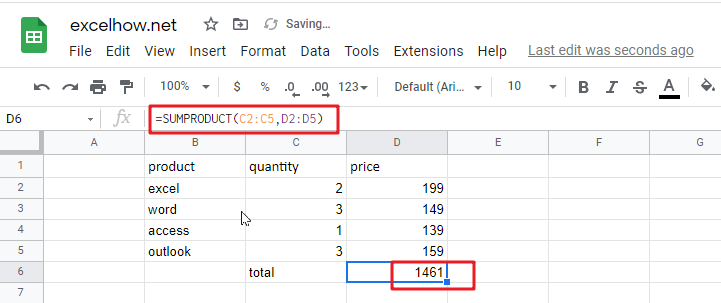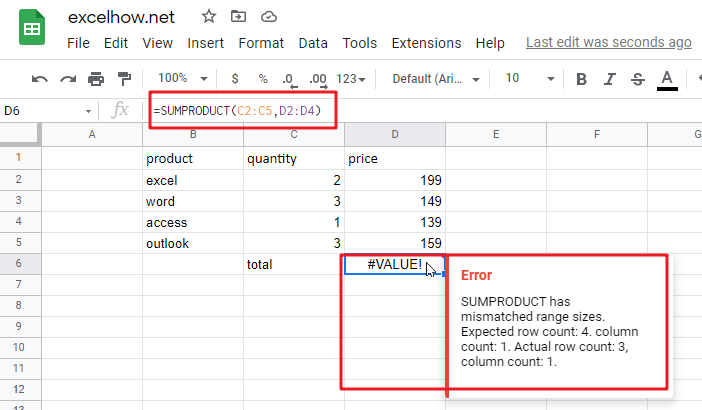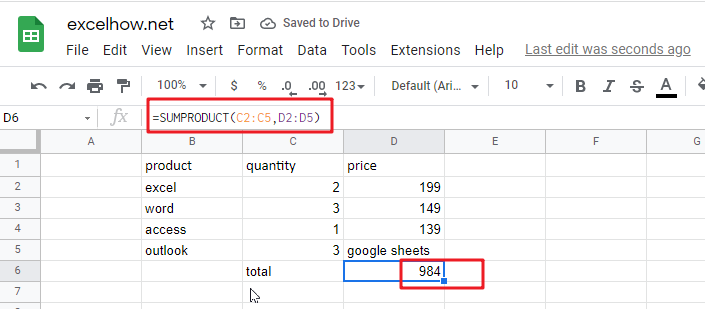# ExcelHow

This post will guide you how to use Google Sheets SUMPRODUCT function with syntax and examples in Google Spreadsheets.

## Description

The Google Sheets SUMPRODUCT function multiplies corresponding components in the given one or more arrays or ranges, and returns the sum of those products. And it returns a numeric value.

The SUMPRODUCT function is a build-in function in Google Spreadsheets and it is categorized as a Array Function.

## Syntax

The syntax of the SUMPRODUCT function is as below:

`= SUMPRODUCT (array1,[array2],…)`

Where the SUMPRODUCT function arguments are:

• `array1 `-This is a required argument. The first array or range that you want to multiply and then add.
• `[array2],`– This is an optional argument.  The second array or range that you want to multiply and then add.

Note:

• The array arguments must have the same dimensions, or it will return the #VALUE! Error.
• The SUMPRODUCT treats non-numeric items in the arrays as zeros.

## Google Sheets SUMPRODUCT Function Example

The below examples will show you how to use Google Sheets SUMPRODUCT Function to return the sum of products.

#1 To get the total amount of all products in the range B1:B5, just using the following Google Sheets formula:

`=SUMPRODUCT(C2:C5,D2:D5)`2# The arrays or ranges must have the same dimensions, otherwise returns the #VALUE error. See the below picture:3# non-numeric items in the arrays or ranges are treated as zeros.